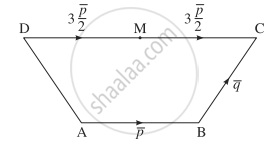# ABCD is a trapezium with AB parallel to DC and DC = 3AB. M is the midpoint of DC. ABpBCqAB¯=p¯,BC¯=q¯.Find in terms of pandqp¯andq¯: (i) AMAM¯ (ii) BDBD¯ (iii) MBMB¯ (iv) DADA¯ - Mathematics and Statistics

Sum

ABCD is a trapezium with AB parallel to DC and DC = 3AB. M is the midpoint of DC. bar"AB" = bar"p", bar"BC" = bar"q".
Find in terms of bar"p" and bar"q":

(i) bar"AM" (ii) bar"BD" (iii) bar "MB" (iv) bar"DA"

#### SolutionDC is parallel to AB and DC = 3AB.

∵ bar"AB" = bar"p"  therefore  bar"DC" = 3bar"p"

M is the midpoint of DC

∴ bar"DM" = bar"MC" = 1/2bar"DC" = 3/2bar"p"

(i) bar"AM" = bar"AM" + bar"BC" + bar"CM"

= bar"AB" + bar"BC" - bar"MC"

= bar"p" + bar"q" - "3p"/2

= bar"q" - 1/2 bar"p"

(ii) bar"BD" = bar"BC" + bar"CD" = bar"BC" - bar"DC" = bar"q" - 3bar"p"

(iii) bar"MB" = bar"MC" + bar"CB" = bar"MC" - bar"BC" = 3/2 bar"p" - bar"q"

(iv) bar"DA" = bar"DC" + bar"CB" + bar"BA" = bar"DC" - bar"BC" - bar"AB"

= 3bar"p" - bar"q" - bar"p" = 2bar"p" - bar"q"

#### Notes

[Note: In the textbook answer instead of p and q, a and b are written.]

Concept: Representation of Vector
Is there an error in this question or solution?